# The plan

The plan of the housing estate is in three scales 1: 5000,1: 10000,1: 15000. The distance between two points on a plan with a scale of 1: 10000 is 12 cm. What is this distance on the other two plans? What is this distance?

x1 =  24 cm
x3 =  8 cm
s2 =  1.2 km

### Step-by-step explanation:Did you find an error or inaccuracy? Feel free to write us. Thank you!Tips to related online calculators
Need help to calculate sum, simplify or multiply fractions? Try our fraction calculator.
Check out our ratio calculator.
Do you want to convert length units?

## Related math problems and questions:

• Scale of the map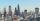The distance between two cities is actually 30 km and the map is 6 cm. What is the scale of the map?
• Plan scale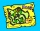The actual width of the room is 66 dm, the width in plan is 4.4 cm. What scale corresponds to the plan?
• Map scaleThe rectangular plot has a scale of 1: 10000 area 3 cm2 on the map. What content does this plot have on a 1:5000 scale map?
• Scale of the mapDetermine the map's scale, which is the actual distance of 120 km l represented by a segment long 6 cm.
• The distance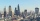The distance between two towns on a given map is 3 ½ cm. If ½ cm represents 6km. , what is the distance between the two towns?
• The scaleThe distance of 15 cm on the map corresponds to a distance of 27 km in reality. What is the scale of the map?
• Map 2At what scale is made map if the distance 8.2 km corresponds on the map segment 5 cm long?
• Two villages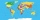Two villages are 11 km and 500 m away. On the map, their distance is determined by a 5 cm long line. Find the scale of the map.
• Geometric plan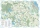At what scale the building plan if one side of the building is 45m long and 12mm long on a plan?
• Map scale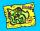What is the scale of the map, if 5.4 km is 72 mm on the map?
• Scale 3Miriam room is 3.2 meters wide. It is draw by line segment length 6.4 cm on floor plan. In what scale it is plan of the room?
• Two villagesOn the map with a scale of 1:40000 are drawn two villages actually 16 km away. What is their distance on the map?
• Small city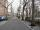The length of the street is 230 m. What will be the length of its image on the plan at a scale of 1: 2500?
• On the mapA line 1.5 cm long corresponds to a line 3 cm long on the map. What is the scale of the map?
• Scale of planOn the plan of the village in the scale of 1: 1000 a rectangular garden is drawn. Its dimensions on the plan are 25mm and 28mm. Determine the area of the garden in ares.
• The sidewalk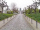The sidewalk is 50 m long. What will be its length on the plot plan in the scale of 1: 200?
• Map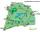On the tourist map at a scale of 1 : 50000 is distance between two points along a straight road 3.7 cm. How long travels this distance on a bike at 30 km/h? Time express in minutes.